# sql求分类跟合计：rollup()函数

select

area_code,

sum(CASE WHEN owner_type = 0 THEN 1 ELSE 0 END) as legalPersonNum,

sum(CASE WHEN owner_type = 1 THEN 1 ELSE 0 END) as adminNum

where area_code is not null and area_code != ''

group by rollup(area_code)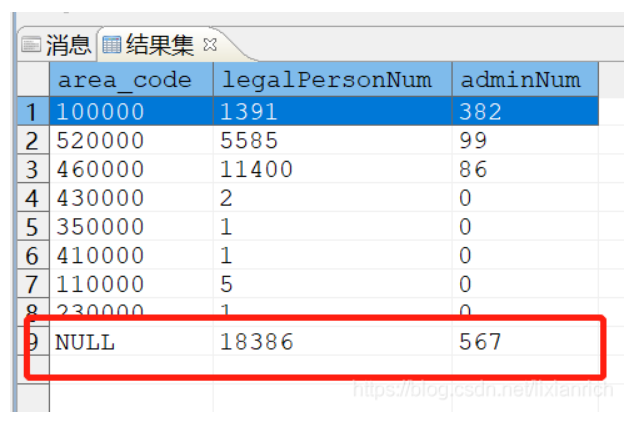1、第一种，采用union

select

'合计' as area_code,sum(legalPersonNum) as legalPersonNum,

from(

select

area_code,

sum(CASE WHEN owner_type = 0 THEN 1 ELSE 0 END) as legalPersonNum,

sum(CASE WHEN owner_type = 1 THEN 1 ELSE 0 END) as adminNum

where area_code is not null and area_code != ''

group by area_code

order by area_code

)

union

select

area_code,

sum(CASE WHEN owner_type = 0 THEN 1 ELSE 0 END) as legalPersonNum,

sum(CASE WHEN owner_type = 1 THEN 1 ELSE 0 END) as adminNum

where area_code is not null and area_code != ''

group by area_code

order by area_code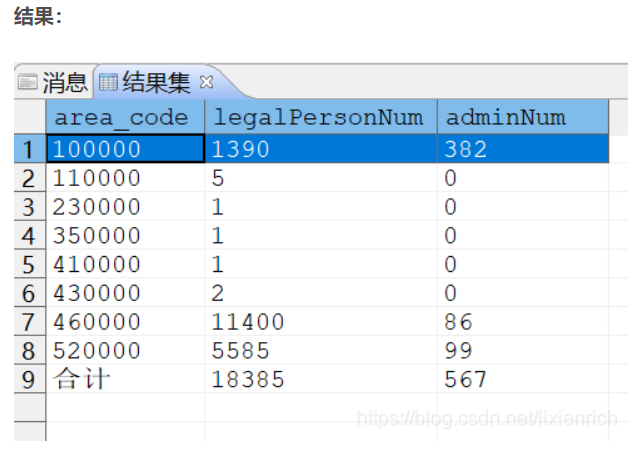2、采用rollup()函数

select

decode(grouping(area_code),1,'total',area_code) as area_code,

sum(CASE WHEN owner_type = 0 THEN 1 ELSE 0 END) as legalPersonNum,

sum(CASE WHEN owner_type = 1 THEN 1 ELSE 0 END) as adminNum

where area_code is not null and area_code != ''

group by rollup(area_code)

order by area_code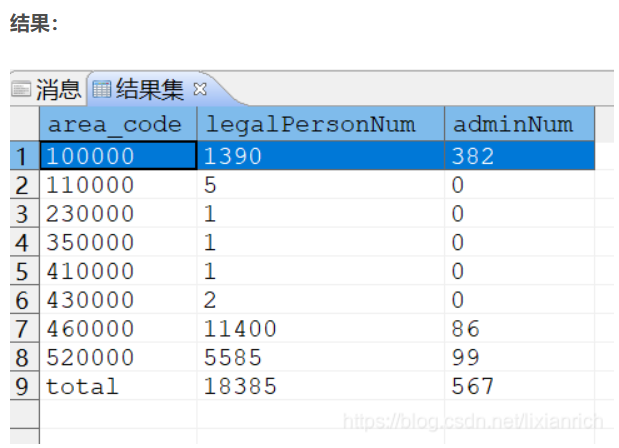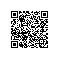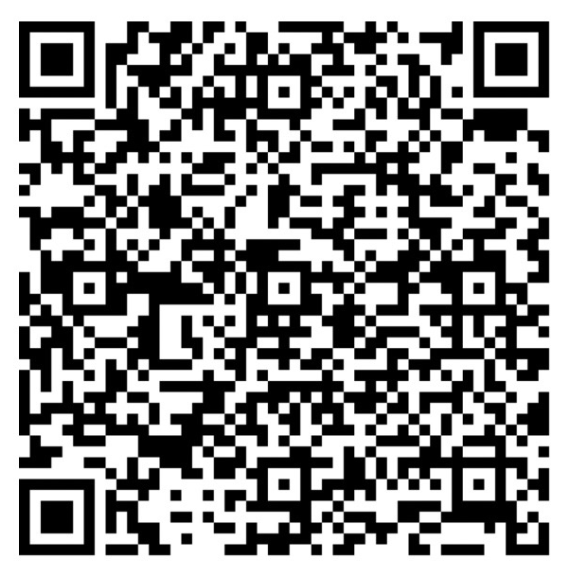使用钉钉扫一扫加入圈子
+ 订阅